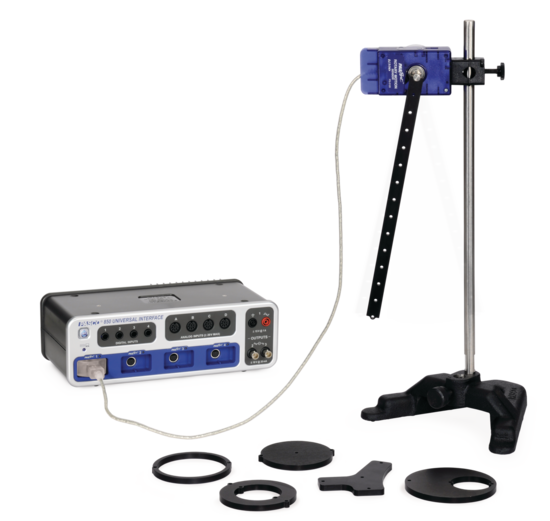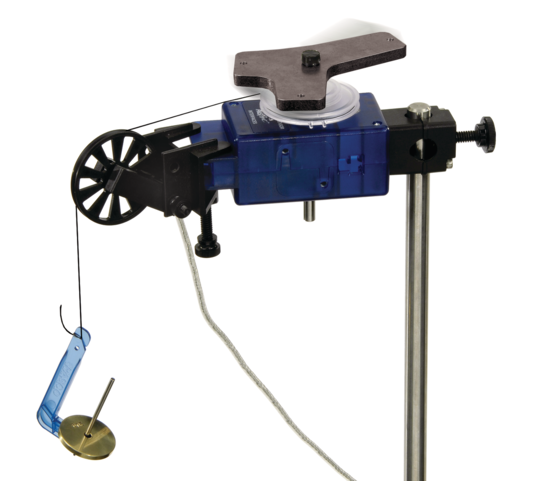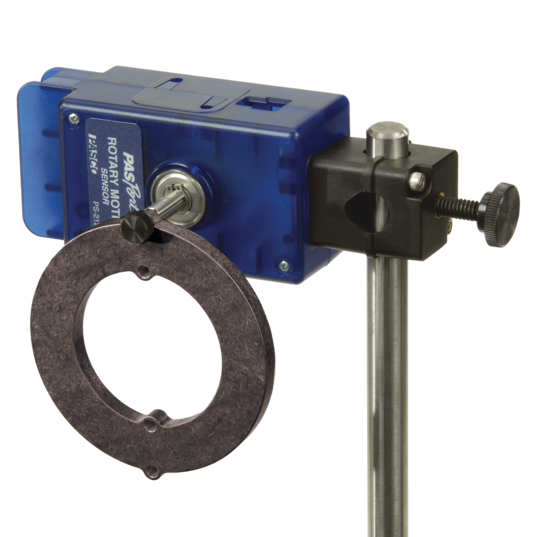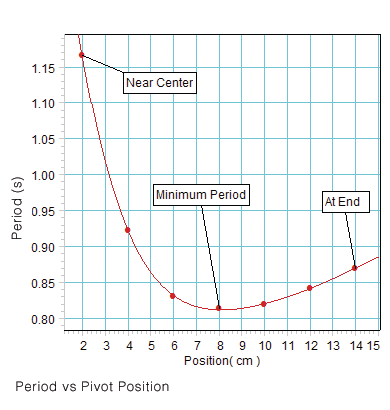Apply a known torque and measure the angular acceleration to calculate the moment of inertia of the object. Multiple holes in the plate allow investigation of the Parallel Axis Theorem.Unique design allows pivot exactly at the edge. Measure the period of the thick ring oscillating at either the inner or outer radius.The Pendulum Bar has holes spaced at 2 cm intervals. A graph of Oscillation Period vs. Pivot Hole Position shows that there is a unique placement that gives a minimum period. This location can be verified using calculus.
U.S. Educator Price
\$410